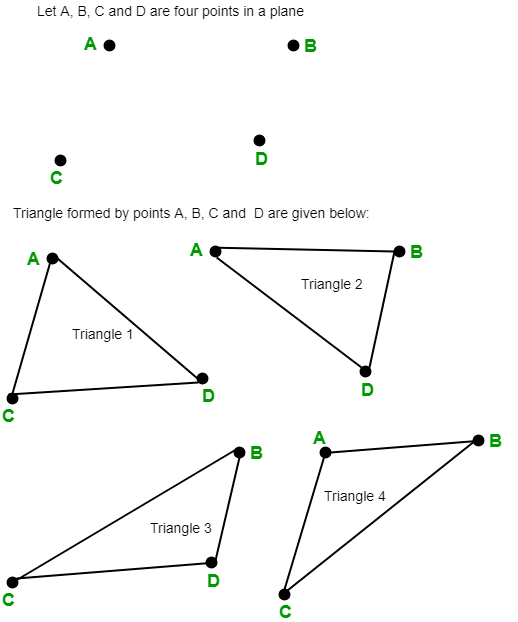GFG App
Open AppBrowser
Continue

# Number of triangles in a plane if no more than two points are collinear

Given n points in a plane and no more than two points are collinear, the task is to count the number of triangles in a given plane.

Examples:

Input :  n = 3
Output : 1

Input :  n = 4
Output : 4Let there are n points in a plane and no three or more points are collinear then number of triangles in the given plane is given by## C++

 // C++ program to find the number of // triangles in a plane if no more // then two points are collinear. #include  using namespace std;   // Function to find number of triangles // in a plane. int countNumberOfTriangles(int n) {       // Formula to find number of triangles     // nC3 = n * (n - 1) * (n - 2) / 6     return n * (n - 1) * (n - 2) / 6; }   // Driver code int main() {     int n = 4;     cout << countNumberOfTriangles(n);     return 0; }

## C

 // C program to find the number of // triangles in a plane if no more // then two points are collinear. #include    // Function to find number of triangles // in a plane. int countNumberOfTriangles(int n) {     // Formula to find number of triangles     // nC3 = n * (n - 1) * (n - 2) / 6     return n * (n - 1) * (n - 2) / 6; }   // Driver code int main() {     int n = 4;     printf("%d",countNumberOfTriangles(n));     return 0; }   // This code is contributed by kothavvsaakash.

## Java

 // Java program to find the number of // triangles in a plane if no more // then two points are collinear. import java.io.*;   class GFG {       // Function to find number of triangles     // in a plane.     static int countNumberOfTriangles(int n)     {           // Formula to find number of triangle         // nC3 = n * (n - 1) * (n - 2) / 6         return n * (n - 1) * (n - 2) / 6;     }       // Driver code     public static void main(String[] args)     {         int n = 4;           System.out.println(             countNumberOfTriangles(n));     } }

## Python3

 # Python3 program to find  # the number of triangles  # in a plane if no more # then two points are collinear.   # Function to find number # of triangles in a plane. def countNumberOfTriangles(n) :           # Formula to find      # number of triangles     # nC3 = n * (n - 1) *     # (n - 2) / 6     return (n * (n - 1) *                 (n - 2) // 6)   # Driver Code if __name__ == '__main__' :           n = 4     print(countNumberOfTriangles(n))                     # This code is contributed # by ajit

## C#

 // C# program to find the  // number of triangles in  // a plane if no more then  // two points are collinear. using System;   class GFG  {       // Function to find number     // of triangles in a plane.     static int countNumberOfTriangles(int n)     {           // Formula to find number          // of triangle          // nC3 = n * (n - 1) *         //           (n - 2) / 6         return n * (n - 1) *                     (n - 2) / 6;     }       // Driver code     public static void Main()     {         int n = 4;           Console.WriteLine(             countNumberOfTriangles(n));     } }   // This code is contributed by anuj_67.

## PHP

 

## Javascript

 

Output:

4

Time complexity: O(1)

Auxiliary space: O(1)

My Personal Notes arrow_drop_up